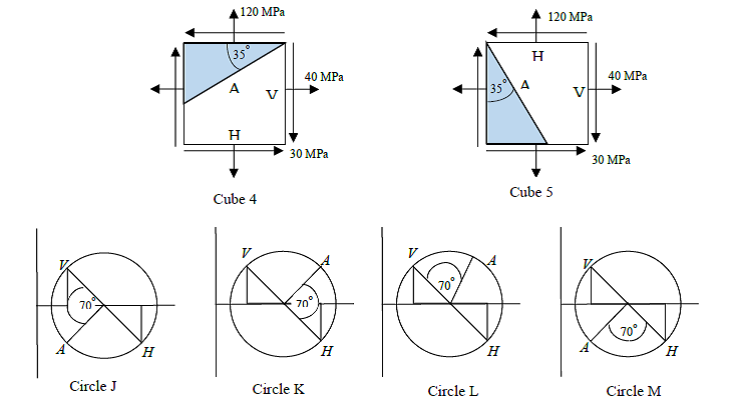# 8.2 Mohr Circle for Stress

Name Email
1. Cube 1  is associated with the
2. Cube 2  is associated with the
3. Cube 3  is associated with the
4.V and H refer to the vertical and horizontal plane, respectively in circle G. The principal angle 1 is
5. V and H refer to the vertical and horizontal plane, respectively in circle H. The principal angle 1 is
6. V and H refer to the vertical and horizontal plane, respectively in circle I. The principal angle 1 is
7.Cube 4 is associated with Mohr’s
8. Cube 5 is associated with Mohr’s
9. The coordinates of the center of the Mohr’s circle corresponding to cube 4 (or cube 5) is
10. The radius of the Mohr’s circle corresponding to cube 4 (or cube 5) is